Documentation

# stem

Plot discrete sequence data

## Syntax

``stem(Y)``
``stem(X,Y)``
``stem(___,'filled')``
``stem(___,LineSpec)``
``stem(___,Name,Value)``
``stem(ax,___)``
``h = stem(___)``

## Description

example

````stem(Y)` plots the data sequence, `Y`, as stems that extend from a baseline along the x-axis. The data values are indicated by circles terminating each stem.If `Y` is a vector, then the x-axis scale ranges from 1 to `length(Y)`.If `Y` is a matrix, then `stem` plots all elements in a row against the same x value, and the x-axis scale ranges from 1 to the number of rows in `Y`.```

example

````stem(X,Y)` plots the data sequence, `Y`, at values specified by `X`. The `X` and `Y` inputs must be vectors or matrices of the same size. Additionally, `X` can be a row or column vector and `Y` must be a matrix with `length(X)` rows.If `X` and `Y` are both vectors, then `stem` plots entries in `Y` against corresponding entries in `X`.If `X` is a vector and `Y` is a matrix, then `stem` plots each column of `Y` against the set of values specified by `X`, such that all elements in a row of `Y` are plotted against the same value. If `X` and `Y` are both matrices, then `stem` plots columns of `Y` against corresponding columns of `X`. ```

example

````stem(___,'filled')` fills the circles. Use this option with any of the input argument combinations in the previous syntaxes.```

example

````stem(___,LineSpec)` specifies the line style, marker symbol, and color. ```

example

````stem(___,Name,Value)` modifies the stem chart using one or more `Name,Value` pair arguments. ```

example

````stem(ax,___)` plots into the axes specified by `ax` instead of into the current axes (`gca`). The option, `ax`, can precede any of the input argument combinations in the previous syntaxes.```

example

````h = stem(___)` returns a vector of `Stem` objects in `h`. Use `h` to modify the stem chart after it is created.```

## Examples

collapse all

Create a stem plot of 50 data values between $-2\pi$ and $2\pi$.

```figure Y = linspace(-2*pi,2*pi,50); stem(Y)```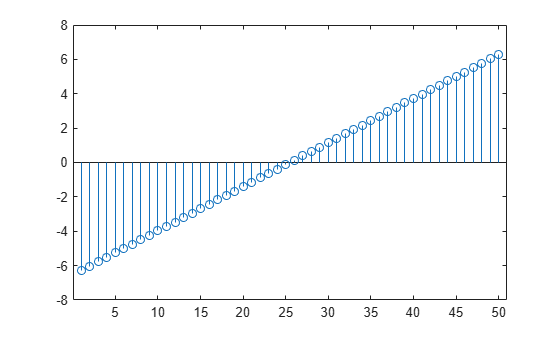Data values are plotted as stems extending from the baseline and terminating at the data value. The length of `Y` automatically determines the position of each stem on the x-axis.

Plot two data series using a two-column matrix.

```figure X = linspace(0,2*pi,50)'; Y = [cos(X), 0.5*sin(X)]; stem(Y)```Each column of `Y` is plotted as a separate series, and entries in the same row of `Y` are plotted against the same x value. The number of rows in `Y` automatically generates the position of each stem on the x-axis.

Plot 50 data values of cosine evaluated between 0 and $2\pi$ and specify the set of x values for the stem plot.

```figure X = linspace(0,2*pi,50)'; Y = cos(X); stem(X,Y)```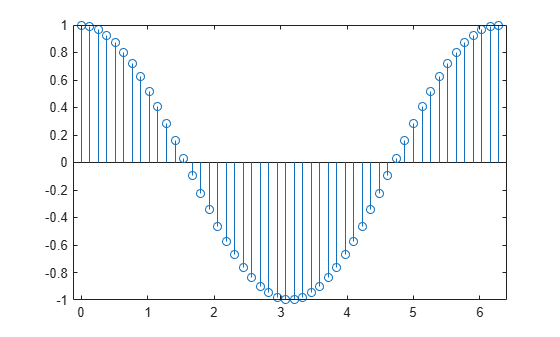The first vector input determines the position of each stem on the x-axis.

Plot 50 data values of sine and cosine evaluated between 0 and $2\pi$ and specify the set of x values for the stem plot.

```figure X = linspace(0,2*pi,50)'; Y = [cos(X), 0.5*sin(X)]; stem(X,Y)```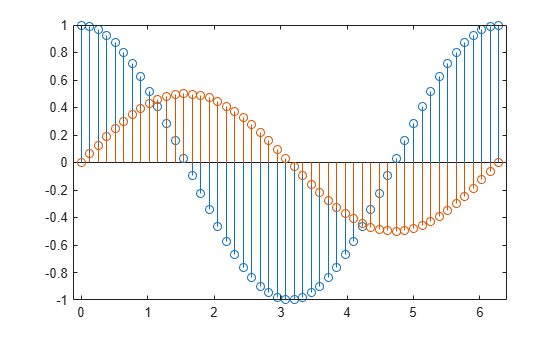The vector input determines the x-axis positions for both data series.

Plot 50 data values of sine and cosine evaluated at different sets of x values. Specify the corresponding sets of x values for each series.

```figure x1 = linspace(0,2*pi,50)'; x2 = linspace(pi,3*pi,50)'; X = [x1, x2]; Y = [cos(x1), 0.5*sin(x2)]; stem(X,Y)```Each column of `X` is plotted against the corresponding column of `Y`.

Create a stem plot and fill in the circles that terminate each stem.

```X = linspace(0,10,20)'; Y = (exp(0.25*X)); stem(X,Y,'filled')```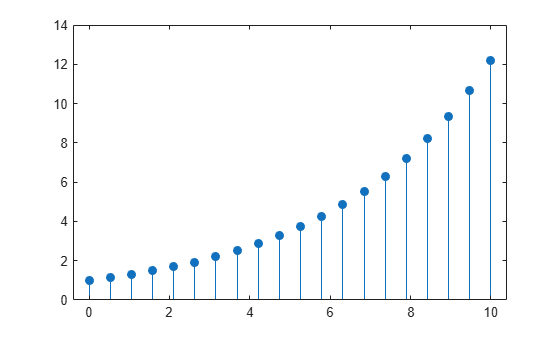Create a stem plot and set the line style to a dotted line, the marker symbols to diamonds, and the color to red using the `LineSpec` option.

```figure X = linspace(0,2*pi,50)'; Y = (exp(X).*sin(X)); stem(X,Y,':diamondr')```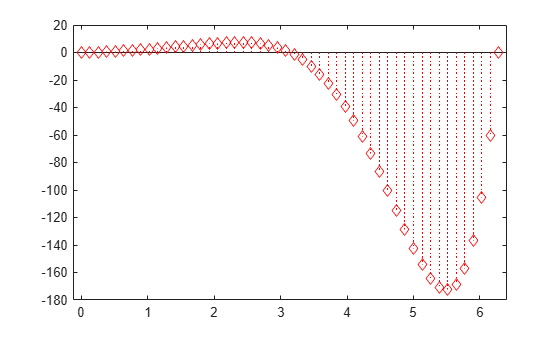To color the inside of the diamonds, use the `'fill'` option.

Create a stem plot and set the line style to a dot-dashed line, the marker face color to red, and the marker edge color to green using `Name,Value` pair arguments.

```figure X = linspace(0,2*pi,25)'; Y = (cos(2*X)); stem(X,Y,'LineStyle','-.',... 'MarkerFaceColor','red',... 'MarkerEdgeColor','green')```The stem remains the default color.

Starting in R2019b, you can display a tiling of plots using the `tiledlayout` and `nexttile` functions. Call the `tiledlayout` function to create a 2-by-1 tiled chart layout. Call the `nexttile` function to create the axes objects `ax1` and `ax2`. Create separate stem plots in the axes by specifying the axes object as the first argument to `stem`.

```x = 0:25; y1 = exp(0.1*x); y2 = -exp(.05*x); tiledlayout(2,1) % Top plot ax1 = nexttile; stem(ax1,x,y1) % Bottom plot ax2 = nexttile; stem(ax2,x,y2)```Create a stem plot.

```X = 0:25; Y = [cos(X); exp(0.05*X)]'; h = stem(X,Y);```The `stem` function creates a stem series object for each column of data. The output argument, `h`, contains the two stem series objects.

Set the first stem series color to green. Change the markers of the second stem series to squares. Starting in R2014b, you can use dot notation to set properties. If you are using an earlier release, use the `set` function instead.

```h(1).Color = 'green'; h(2).Marker = 'square';```Create a stem plot and change properties of the baseline.

```X = linspace(0,2*pi,50); Y = exp(0.3*X).*sin(3*X); h = stem(X,Y);```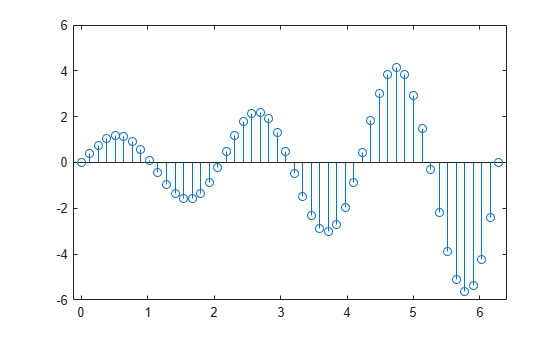Change the line style of the baseline. Starting in R2014b, you can use dot notation to set properties. If you are using an earlier release, use the `set` function instead.

```hbase = h.BaseLine; hbase.LineStyle = '--';```Hide the baseline by setting its `Visible` property to `'off'` .

`hbase.Visible = 'off';`Create a stem plot with a baseline level at 2.

```X = linspace(0,2*pi,50)'; Y = (exp(0.3*X).*sin(3*X)); stem(X,Y,'BaseValue',2);```## Input Arguments

collapse all

Data sequence to display, specified as a vector or matrix. When `Y` is a vector, `stem` creates one `Stem` object. When `Y` is a matrix, `stem` creates a separate `Stem` object for each column.

Data Types: `single` | `double` | `int8` | `int16` | `int32` | `int64` | `uint8` | `uint16` | `uint32` | `uint64` | `categorical` | `datetime` | `duration`

Locations to plot data values in `Y`, specified as a vector or matrix. When `Y` is a vector, `X` must be a vector of the same size. When `Y` is a matrix, `X` must be a matrix of the same size, or a vector whose length equals the number of rows in `Y`.

Data Types: `single` | `double` | `int8` | `int16` | `int32` | `int64` | `uint8` | `uint16` | `uint32` | `uint64` | `categorical` | `datetime` | `duration`

Line style, marker, and color, specified as a character vector or string. For more information, see `LineSpec`.

Example: `':*r'`

`Axes` object. If you do not specify the axes, then `stem` plots into the current axes.

### Name-Value Pair Arguments

Specify optional comma-separated pairs of `Name,Value` arguments. `Name` is the argument name and `Value` is the corresponding value. `Name` must appear inside quotes. You can specify several name and value pair arguments in any order as `Name1,Value1,...,NameN,ValueN`.

Example: `'LineStyle',':','MarkerFaceColor','red'` plots the stem as a dotted line and colors the marker face red.

The `Stem` properties listed here are only a subset. For a complete list, see Stem Properties.

Line style, specified as one of the options listed in this table.

Line StyleDescriptionResulting Line
`'-'`Solid line`'--'`Dashed line`':'`Dotted line`'-.'`Dash-dotted line`'none'`No lineNo line

Line width, specified as a positive value in points, where 1 point = 1/72 of an inch. If the line has markers, then the line width also affects the marker edges.

Stem color, specified as an RGB triplet, a hexadecimal color code, a color name, or a short name.

For a custom color, specify an RGB triplet or a hexadecimal color code.

• An RGB triplet is a three-element row vector whose elements specify the intensities of the red, green, and blue components of the color. The intensities must be in the range `[0,1]`; for example, ```[0.4 0.6 0.7]```.

• A hexadecimal color code is a character vector or a string scalar that starts with a hash symbol (`#`) followed by three or six hexadecimal digits, which can range from `0` to `F`. The values are not case sensitive. Thus, the color codes `'#FF8800'`, `'#ff8800'`, `'#F80'`, and `'#f80'` are equivalent.

Alternatively, you can specify some common colors by name. This table lists the named color options, the equivalent RGB triplets, and hexadecimal color codes.

Color NameShort NameRGB TripletHexadecimal Color CodeAppearance
`'red'``'r'``[1 0 0]``'#FF0000'``'green'``'g'``[0 1 0]``'#00FF00'``'blue'``'b'``[0 0 1]``'#0000FF'``'cyan'` `'c'``[0 1 1]``'#00FFFF'``'magenta'``'m'``[1 0 1]``'#FF00FF'``'yellow'``'y'``[1 1 0]``'#FFFF00'``'black'``'k'``[0 0 0]``'#000000'``'white'``'w'``[1 1 1]``'#FFFFFF'``'none'`Not applicableNot applicableNot applicableNo color

Here are the RGB triplets and hexadecimal color codes for the default colors MATLAB® uses in many types of plots.

`[0 0.4470 0.7410]``'#0072BD'``[0.8500 0.3250 0.0980]``'#D95319'``[0.9290 0.6940 0.1250]``'#EDB120'``[0.4940 0.1840 0.5560]``'#7E2F8E'``[0.4660 0.6740 0.1880]``'#77AC30'``[0.3010 0.7450 0.9330]``'#4DBEEE'``[0.6350 0.0780 0.1840]``'#A2142F'`Example: `'blue'`

Example: ```[0 0 1]```

Example: `'#0000FF'`

Marker symbol, specified as one of the markers listed in this table.

ValueDescription
`'o'`Circle
`'+'`Plus sign
`'*'`Asterisk
`'.'`Point
`'x'`Cross
`'square'` or `'s'`Square
`'diamond'` or `'d'`Diamond
`'^'`Upward-pointing triangle
`'v'`Downward-pointing triangle
`'>'`Right-pointing triangle
`'<'`Left-pointing triangle
`'pentagram'` or `'p'`Five-pointed star (pentagram)
`'hexagram'` or `'h'`Six-pointed star (hexagram)
`'none'`No markers

Example: `'+'`

Example: `'diamond'`

Marker size, specified as a positive value in points, where 1 point = 1/72 of an inch.

Marker outline color, specified as `'auto'`, an RGB triplet, a hexadecimal color code, a color name, or a short name. The default value of `'auto'` uses the same color as the `Color` property.

For a custom color, specify an RGB triplet or a hexadecimal color code.

• An RGB triplet is a three-element row vector whose elements specify the intensities of the red, green, and blue components of the color. The intensities must be in the range `[0,1]`; for example, ```[0.4 0.6 0.7]```.

• A hexadecimal color code is a character vector or a string scalar that starts with a hash symbol (`#`) followed by three or six hexadecimal digits, which can range from `0` to `F`. The values are not case sensitive. Thus, the color codes `'#FF8800'`, `'#ff8800'`, `'#F80'`, and `'#f80'` are equivalent.

Alternatively, you can specify some common colors by name. This table lists the named color options, the equivalent RGB triplets, and hexadecimal color codes.

Color NameShort NameRGB TripletHexadecimal Color CodeAppearance
`'red'``'r'``[1 0 0]``'#FF0000'``'green'``'g'``[0 1 0]``'#00FF00'``'blue'``'b'``[0 0 1]``'#0000FF'``'cyan'` `'c'``[0 1 1]``'#00FFFF'``'magenta'``'m'``[1 0 1]``'#FF00FF'``'yellow'``'y'``[1 1 0]``'#FFFF00'``'black'``'k'``[0 0 0]``'#000000'``'white'``'w'``[1 1 1]``'#FFFFFF'``'none'`Not applicableNot applicableNot applicableNo color

Here are the RGB triplets and hexadecimal color codes for the default colors MATLAB uses in many types of plots.

`[0 0.4470 0.7410]``'#0072BD'``[0.8500 0.3250 0.0980]``'#D95319'``[0.9290 0.6940 0.1250]``'#EDB120'``[0.4940 0.1840 0.5560]``'#7E2F8E'``[0.4660 0.6740 0.1880]``'#77AC30'``[0.3010 0.7450 0.9330]``'#4DBEEE'``[0.6350 0.0780 0.1840]``'#A2142F'`Marker fill color, specified as `'auto'`, an RGB triplet, a hexadecimal color code, a color name, or a short name. The `'auto'` option uses the same color as the `Color` property of the parent axes. If you specify `'auto'` and the axes plot box is invisible, the marker fill color is the color of the figure.

For a custom color, specify an RGB triplet or a hexadecimal color code.

• An RGB triplet is a three-element row vector whose elements specify the intensities of the red, green, and blue components of the color. The intensities must be in the range `[0,1]`; for example, ```[0.4 0.6 0.7]```.

• A hexadecimal color code is a character vector or a string scalar that starts with a hash symbol (`#`) followed by three or six hexadecimal digits, which can range from `0` to `F`. The values are not case sensitive. Thus, the color codes `'#FF8800'`, `'#ff8800'`, `'#F80'`, and `'#f80'` are equivalent.

Alternatively, you can specify some common colors by name. This table lists the named color options, the equivalent RGB triplets, and hexadecimal color codes.

Color NameShort NameRGB TripletHexadecimal Color CodeAppearance
`'red'``'r'``[1 0 0]``'#FF0000'``'green'``'g'``[0 1 0]``'#00FF00'``'blue'``'b'``[0 0 1]``'#0000FF'``'cyan'` `'c'``[0 1 1]``'#00FFFF'``'magenta'``'m'``[1 0 1]``'#FF00FF'``'yellow'``'y'``[1 1 0]``'#FFFF00'``'black'``'k'``[0 0 0]``'#000000'``'white'``'w'``[1 1 1]``'#FFFFFF'``'none'`Not applicableNot applicableNot applicableNo color

Here are the RGB triplets and hexadecimal color codes for the default colors MATLAB uses in many types of plots.

`[0 0.4470 0.7410]``'#0072BD'``[0.8500 0.3250 0.0980]``'#D95319'``[0.9290 0.6940 0.1250]``'#EDB120'``[0.4940 0.1840 0.5560]``'#7E2F8E'``[0.4660 0.6740 0.1880]``'#77AC30'``[0.3010 0.7450 0.9330]``'#4DBEEE'``[0.6350 0.0780 0.1840]``'#A2142F'`## Output Arguments

collapse all

`Stem` objects. These are unique identifiers, which you can use to modify the properties of a specific `Stem` object after it is created.